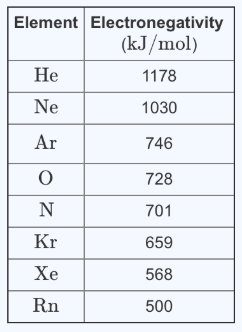# Problem: After doing some analysis, the probe determines that the gas pocket contains an element that has an ionization energy of I = 1527 kJ/mol and an electron affinity of EA = 33.0 kJ/mol. What is the identity of this element?Express your answer using an element symbol (e.g., Hg).As part of the exploration of one of the moons of Jupiter, a probe digs under the surface, where it finds a pocket of gas. It starts to do some on-board experimentation. The data stored in the probe's computer include the following table of Mulliken electronegativity values, χ, of gases:The definition of Mulliken electronegativityThe electronegativity of an element has been defined by Mulliken according to the expressionχ=(I-EA)2where χ is the Mulliken electronegativity, I is the ionization energy, and EA is the electron affinity.

###### FREE Expert Solution
90% (188 ratings)View Complete Written Solution
###### Problem Details

After doing some analysis, the probe determines that the gas pocket contains an element that has an ionization energy of I = 1527 kJ/mol and an electron affinity of EA = 33.0 kJ/mol. What is the identity of this element?

As part of the exploration of one of the moons of Jupiter, a probe digs under the surface, where it finds a pocket of gas. It starts to do some on-board experimentation. The data stored in the probe's computer include the following table of Mulliken electronegativity values, χ, of gases:The definition of Mulliken electronegativity

The electronegativity of an element has been defined by Mulliken according to the expression

$\mathbf{\chi }\mathbf{=}\frac{\left(I-\mathrm{EA}\right)}{\mathbf{2}}$

where χ is the Mulliken electronegativity, I is the ionization energy, and EA is the electron affinity.